Word Clock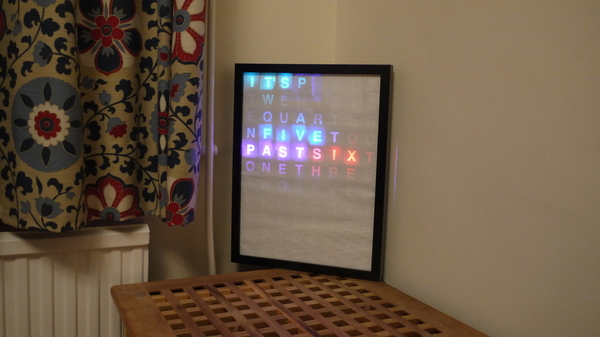This is an LED word clock. It light up letters in a grid to spell out the time.

In order to use a sensible number of LEDs and keep the cost down, the clock will only tell the time to the nearest 5 minutes...

You'll Need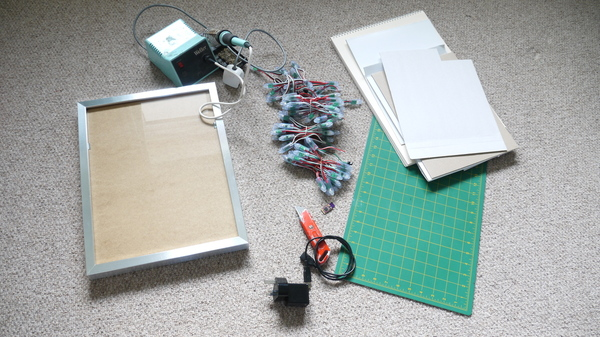• A deep picture frame (we used an Ikea one)
• 88 WS2811 LED lights - for an 8 x 11 grid
• Thick card and tools to cut it
• An Espruino board. Either a Pico, revision 1v4, or a 1v3 with a Clock crystal
• A USB power supply
• Tools for soldering

Assembly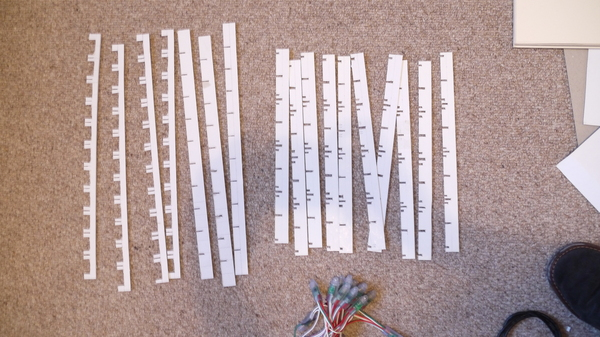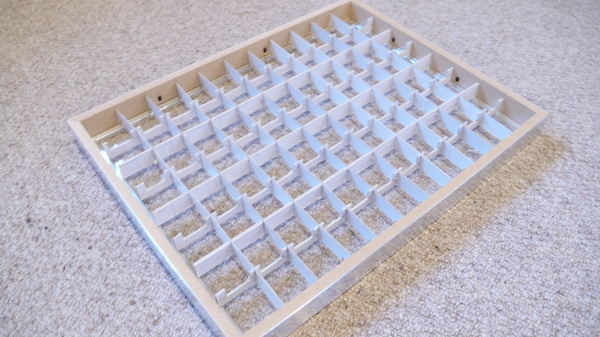Measure and cut the card into 10 x 7 strips as above (measure them to fit the picture frame that you have), and assemble them.

We've left gaps for the WS2811 LEDs and their wires- however we used a string of LEDs. If you buy your LEDs in flat strip you won't need to cut out any large gaps.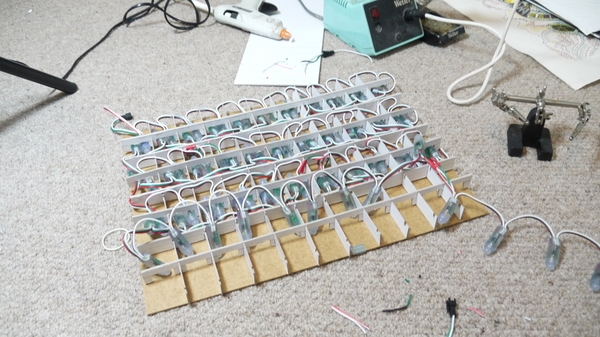Fit in the LEDs in an 'S' shape, and glue them securely with hot glue. Make sure know which end of the LED chain is the input and which is the output! Consult the WS2811 page if you're not sure.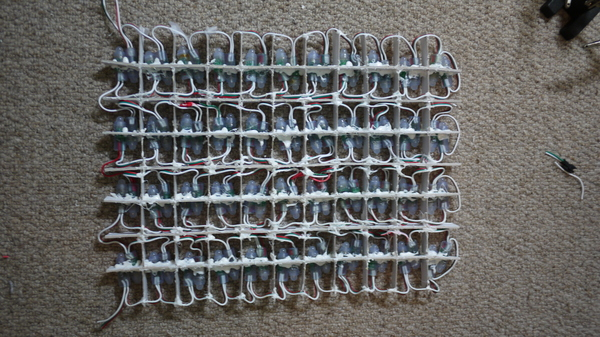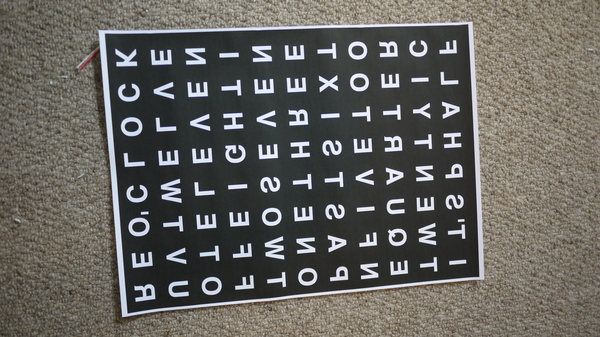Print out a pattern of letters that matches your grid. We did this on plan paper and mirrored it, so that the white paper faces the viewer. However you could do it the other way, or could use acetate and a diffuser.

If you print out the text as we've done, try and do it on a laser printer or photocopier. Printing lots of black on thin paper with a normal inkjet printer will cause it to warp slightly and look ugly.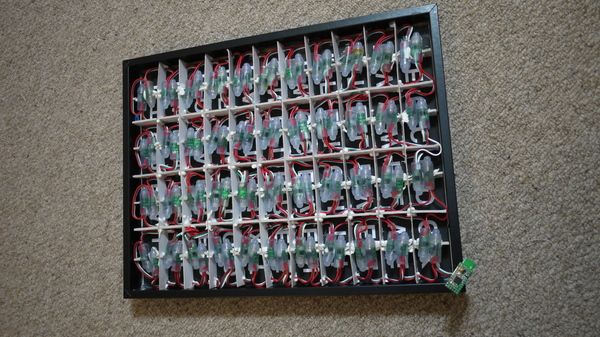Now assemble everything, and wire up to the Espruino board as follows:

Wire Function Espruino
Red LED + Bat
White LED - GND
Green LED data B15

And you're done! On to the software...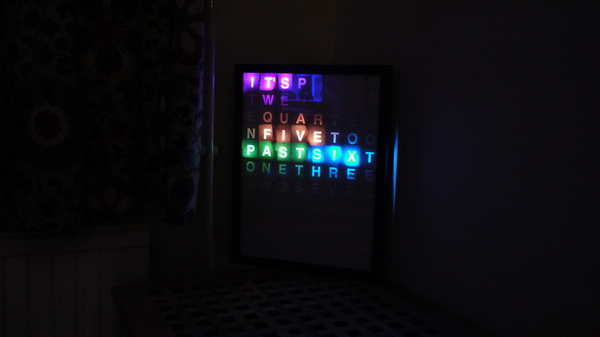Software

Load the code below into Espruino, type save(), and you're sorted!

To set the time, you'll have to modify the 'h', 'm' and 's' variables (hour, minute, and second).

// The indices of each word
var words = {
"its":[76,77,54],
"_five":[80,51,58,29],
"_ten":[75,74,73],
"_quarter":[79,52,57,30,35,8,13],
"_twenty":[75,78,53,56,31,34],
"_half":[32,33,10,11],

"past":[72,81,50,59],
"to":[36,7],

"one":[71,82,49],
"two":[70,83,48],
"three":[60,27,38,5,16],
"four":[69,68,67,66],
"five":[84,85,86,87],
"six":[28,37,6],
"seven":[61,26,39,4,17],
"eight":[47,62,25,40,3],
"nine":[17,18,19,20],
"ten":[15,16,17],
"eleven":[46,63,24,41,2,19],
"twelve":[45,64,23,42,1,20],
"oclock":[44,65,22,43,0,21],

"pico" : [55,9,12,14]
};
// set up SPI
SPI2.setup({baud:3200000, mosi:B15});
var arr = new Uint8Array(8*11*3);
var pos = 0;

// Hue to RGB - we use this to work out what colour to set each word
function HSVtoRGB(h, s, v) {
var r, g, b, i, f, p, q, t;
if (h && s === undefined && v === undefined) {
s = h.s, v = h.v, h = h.h;
}
i = Math.floor(h * 6);
f = h * 6 - i;
p = v * (1 - s);
q = v * (1 - f * s);
t = v * (1 - (1 - f) * s);
switch (i % 6) {
case 0: r = v, g = t, b = p; break;
case 1: r = q, g = v, b = p; break;
case 2: r = p, g = v, b = t; break;
case 3: r = p, g = q, b = v; break;
case 4: r = t, g = p, b = v; break;
case 5: r = v, g = p, b = q; break;
}
return [Math.floor(r * 255),Math.floor(g * 255),Math.floor(b * 255)];
}

// Given an array of words, set the relevant LEDs to light up
// in rainbow colours
function getPattern(wordList) {
arr.fill(0);
var i = 0;
pos+=0.05;
wordList.forEach(function(word) {
words[word].forEach(function(led) {
arr.set(HSVtoRGB(i+pos,1,1,1),led*3);
});
i+=0.2;
});
}

// Convert hours and minutes (seconds are ignored) into a string such as '_five minutes past six'
// We prefix the first part with an underscore so we don't accidentally light the wrong 'five' up
function timeToWords(h,m,s) {
var mins = ["","_five","_ten","_quarter","_twenty","_twenty _five","_half"];
var hours = ["", "one","two","three","four","five","six","seven","eight","nine","ten","eleven","twelve"];

var str = "its ";
// the nearest 5 minutes
var midx = Math.round(m/5);
var hidx = h;
// work out if we're 'past' or 'to' and adjust hour accordingly
if (midx>6) {
if (midx==12) midx=0;
hidx++;
if (hidx>12) hidx -= 12;
}
// finally output minutes and hours
if (midx!=0) {
if (midx<=6)
str += mins[midx]+" past ";
else {
str += mins[12-midx]+" to ";
}
str += hours[hidx];
} else {
str += hours[hidx];
str += " oclock";
}
// print it out for debugging
console.log(h+":"+m+":"+s+"    "+str);
return str;
}

// Very simplistic timekeeping...
var h=5,m=59,s=0;
setInterval(function() {
// increment seconds
s++;
if (s>=60) {
// if seconds goes to 60, inc minutes, and so on...
s=0;
m+=1;
if (m>=60) {
m=0;
h++;
if (h>12)
h=1;
}
}
// Get the time as a string
var timeWords = timeToWords(h,m,s);
// convert it to an array of words and light up the LEDs
getPattern(timeWords.split(" "));
SPI2.send4bit(arr, 0b0001, 0b0011);
}, 1000);

You could also add a way to use buttons to set the time, or you could even use some Internet connectivity to get the time off the net, or GPS to get it from the Global Positioning System.

This page is auto-generated from GitHub. If you see any mistakes or have suggestions, please let us know.